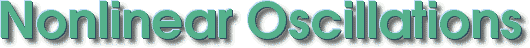An international mathematical journal

# Scope

The journal Nonlinear Oscillations publishes papers on the following topics:

• General problems of oscillation theory and nonlinear mechanics
• Multifrequency oscillations theory
• Asymptotic, iterative, and other approximate methods for solving differential equations
• Qualitative methods of studying differential equations
• Delay differential equations, equations with impulses and random perturbations
• Theory of boundary value problems for ordinary differential and functional differential equations
• Applications

© Institute of Mathematics of the NAS of Ukraine. Last updated 11 May 2022 at 0:28. Contact address: nosc@imath.kiev.ua